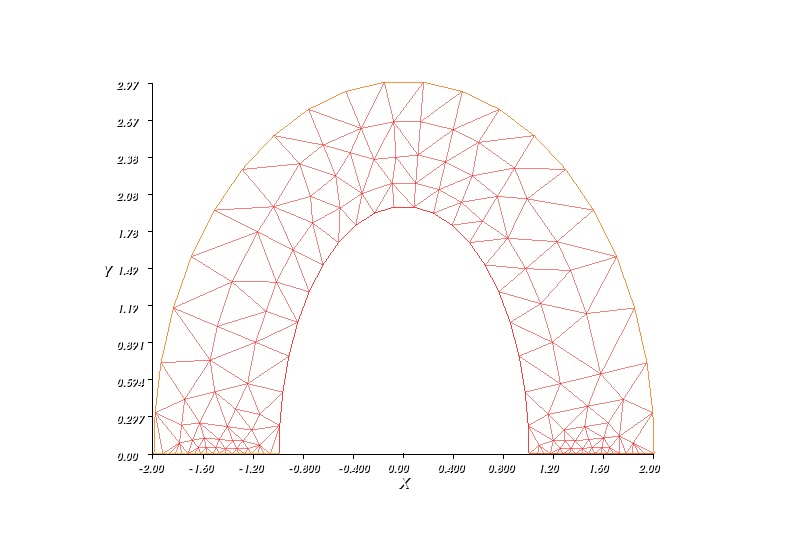# Elasticity Simulation with FreeFEM

## The Problem

This blog continues the Finite Element Methods series for some simple physical phenomena simulation. Previously, I have covered the heat equation. In this post, I want to discuss another famous problem: Elasticity. Concretely, we want to know how object of material deforms under pressure or external force. I will first state the system equation of elasticity before I try to explain what it means (I come from mathematics background, so many concepts in physics appear a little confusing to me) \begin{align} -div(Ae(u)) & = f \tag{1} \\ u_{| \Gamma_D} & = 0 \tag{2} \\ (Ae(u)).n_{| \Gamma_N} & = g \tag{3}\\ \end{align}

Denote

$A \psi = 2 \mu \psi + \lambda (\text{Tr}(\psi)) I_d ,$

and

$e(u) = \frac{1}{2} (\nabla u^T + \nabla u) ,$

where $\mu$ and $\lambda$ are call Lam$'e$ parameters and are defined as follow:

$\mu = \frac{E}{2(1 + \nu)} \\ \lambda = \frac{E\nu}{(1+\nu)(1 - 2\nu)}$

$E$ is Young’s modulus which represents for the stiffness of solid material, and $\nu$ is called Poisson’s ratio which explains for the phenomenon where the material extends in perpendicular with respect to direction of the force. $u$ in the system of equation is the direction for which each particle in the object will move under force $f$ and external force on $g$ some boundary $\Gamma_N$. In fact, I do not really understand why equation (1) is true, but as far as I know the simulation I run on my computer seems right :). One final note before we are going to construct the weak formulation for this problem is the meaning for the boundary conditions. Dirichlet condition for this type of equations is always set to be 0, which means that boundary is kept fixed, which Neumann condition is the external force apply on the object’s edges.

### How to construct weak solution ?

In finite element method, we want to approximate $u$ by basis functions which are defined over all elements of the domain, i.e

$u = \sum_{i = 1}^k \alpha_i \phi_i,$

where $\phi_i$ is basis function corresponding to element $i$. The reason why we want to construct the original problem in the form of $\alpha(u, v) = L(v)$ (*) is that in this case, finding coefficient $\alpha_i$ is equivalent to solving a system of linear equations. Finding $u$ which satisfies (*) for every $v$ in the chosen function space (approximated by span {$\phi_1, \phi_2, …, \phi_k$} is equivalent to finding $u$ which satisfies $\alpha(u, \phi_i) = L(\phi_i)$ for all basis function $\phi_i$. Hence, in its core, we need to solve the following system of equations:

$\forall i \in \{1, 2, ... , k \} \\ \sum_{j = 1}^k \alpha_j \alpha(\phi_j, \phi_i) = L(\phi_i)$

Now, we turn back to our system of elasticity. Denote $v$ as test function and belongs to $[H^1_0 (\Omega)]^2$ (we are working on two dimensional case). The space of test function is usually chosen so that it satisfies the Dirichlet boundary condition. Multiply (1) by $v$ and taking integral on domain $\Omega$, we have:

\begin{align} \int_{\Omega}-div(Ae(u)) & = \int_{\Omega}fv \\ \int_{\Omega} Ae(u) : \nabla v & = \int_{\Omega} fv + \int_{\Gamma_N} gv \end{align}

### Numerical solution

The following code is to simulate how a elliptic shape deform under gravity.

real E = 21e5;
real nu = 0.28;
real f = -1e4;
// Mesh
border C1(t = pi, 0){x = cos(t); y=2*sin(t);}
border C2(t = 0, 1){x = 1+t; y = 0;}
border C3(t = 0, pi){x = 2*cos(t); y = 3*sin(t);}
border C4(t = 0, 1){x = -2+t; y = 0;}
int n = 20;
mesh Th = buildmesh(C1(n) + C2(n) + C3(n) + C4(n));

plot(Th);
// Fespace
fespace Vh(Th, P2);
Vh u, v;
Vh uu, vv;

// Macro
real sqrt2=sqrt(2.);
macro epsilon(u1,u2) [dx(u1),dy(u2),(dy(u1)+dx(u2))/sqrt2] //
macro div(u,v) ( dx(u)+dy(v) ) //

// Problem
real mu= E/(2*(1+nu));
real lambda = E*nu/((1+nu)*(1-2*nu));
real T = 10;
real dt = 0.1;
int count = 0;
for(real t = 0; t <= T; t+=dt) {
solve lame([u, v], [uu, vv])
= int2d(Th)( lambda * div(u, v) * div(uu, vv)
+ 2.*mu * (epsilon(u,v)' * epsilon(uu,vv)) ) -
int2d(Th)( f*vv )
+ on(C2, C4, u=0, v=0);
count ++;
// Plot
real coef= 0.7;
// Move mesh
Th = movemesh(Th, [x+u*coef, y+v*coef]);
plot(Th, ps="./elastic simulation/sol_"+count+".jpg");
}Elastic simulation result